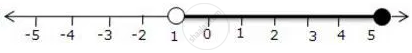Share

# For Graph Given Write an Inequation Taking X as the Variable 4 - Mathematics

Course

#### Question

For graph given write an inequation taking x as the variable#### Solution

-1 < x <= 5, x in R

Is there an error in this question or solution?

#### APPEARS IN

Selina Solution for Concise Mathematics for Class 10 ICSE (2020 (Latest))
Chapter 4: Linear Inequations (In one variable)
Exercise 4(B) | Q: 2.4 | Page no. 49
Solution For Graph Given Write an Inequation Taking X as the Variable 4 Concept: Linear Inequations in One Variable.
S